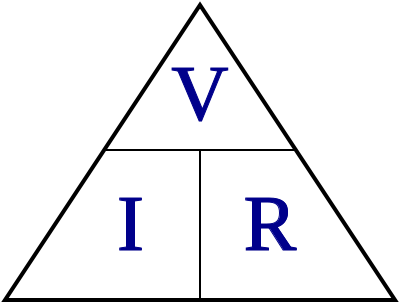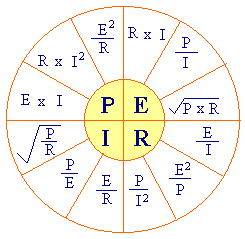# Ohm CalculatorThe relationship between electrical parameters Electrical voltage V, amperage I, resistivity R, impedance Z, wattage or power P

Enter any of the following parameters and rest would be calculated automatically for you.

Ohm's law states that the current through a conductor between two points is directly proportional to the potential difference across the two points. Introducing the constant of proportionality, the resistance, one arrives at the usual mathematical equation that describes this relationship:

Voltage or volts E or V = Volts V

Amperage or current I = amperes, amps A

Resistivity or resistance r= ohms O

Wattage or Power P = watts W

For R take impedance Z

# Power CalculatorThe relationship between electrical parameters Electrical Power P, amperage I and Voltage V

Enter any of the two parameters below and rest would be calculated automatically for you.

In physics, power is the rate at which energy is transferred, used, or transformed. For example, the rate at which a light bulb transforms electrical energy into heat and light is measured in wattsthe more wattage, the more power, or equivalently the more electrical energy is used per unit time.

Electrical power P: watts

Voltage V: Volts,

Amperage I: Amps

For R take impedance Z